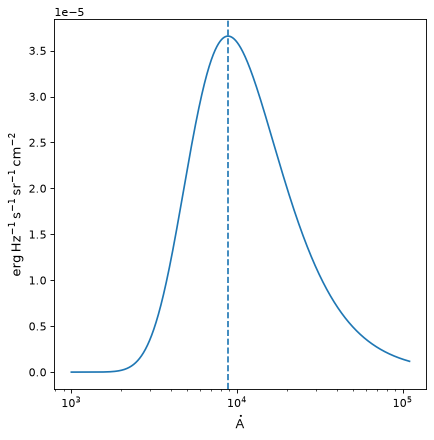# BlackBody¶

class astropy.modeling.physical_models.BlackBody(*args, **kwargs)[source]

Blackbody model using the Planck function.

Parameters
temperature

Blackbody temperature.

scale

Scale factor. If dimensionless, input units will assumed to be in Hz and output units in (erg / (cm ** 2 * s * Hz * sr). If not dimensionless, must be equivalent to either (erg / (cm ** 2 * s * Hz * sr) or erg / (cm ** 2 * s * AA * sr), in which case the result will be returned in the requested units and the scale will be stripped of units (with the float value applied).

Notes

Model formula:

$B_{\nu}(T) = A \frac{2 h \nu^{3} / c^{2}}{exp(h \nu / k T) - 1}$

Examples

>>> from astropy.modeling import models
>>> from astropy import units as u
>>> bb = models.BlackBody(temperature=5000*u.K)
>>> bb(6000 * u.AA)
<Quantity 1.53254685e-05 erg / (cm2 Hz s sr)>

import numpy as np
import matplotlib.pyplot as plt

from astropy.modeling.models import BlackBody
from astropy import units as u
from astropy.visualization import quantity_support

bb = BlackBody(temperature=5778*u.K)
wav = np.arange(1000, 110000) * u.AA
flux = bb(wav)

with quantity_support():
plt.figure()
plt.semilogx(wav, flux)
plt.axvline(bb.nu_max.to(u.AA, equivalencies=u.spectral()).value, ls='--')
plt.show()


(png, svg, pdf)Attributes Summary

 bolometric_flux Bolometric flux. input_units This property is used to indicate what units or sets of units the evaluate method expects, and returns a dictionary mapping inputs to units (or None if any units are accepted). input_units_equivalencies lambda_max Peak wavelength when the curve is expressed as power density. nu_max Peak frequency when the curve is expressed as power density. param_names Names of the parameters that describe models of this type. scale temperature

Methods Summary

 evaluate(x, temperature, scale) Evaluate the model.

Attributes Documentation

bolometric_flux

Bolometric flux.

input_units
input_units_equivalencies = {'x': [(Unit("m"), Unit("Hz"), <function spectral.<locals>.<lambda>>), (Unit("m"), Unit("J"), <function spectral.<locals>.<lambda>>), (Unit("Hz"), Unit("J"), <function spectral.<locals>.<lambda>>, <function spectral.<locals>.<lambda>>), (Unit("m"), Unit("1 / m"), <function spectral.<locals>.<lambda>>), (Unit("Hz"), Unit("1 / m"), <function spectral.<locals>.<lambda>>, <function spectral.<locals>.<lambda>>), (Unit("J"), Unit("1 / m"), <function spectral.<locals>.<lambda>>, <function spectral.<locals>.<lambda>>), (Unit("1 / m"), Unit("rad / m"), <function spectral.<locals>.<lambda>>, <function spectral.<locals>.<lambda>>), (Unit("m"), Unit("rad / m"), <function spectral.<locals>.<lambda>>), (Unit("Hz"), Unit("rad / m"), <function spectral.<locals>.<lambda>>, <function spectral.<locals>.<lambda>>), (Unit("J"), Unit("rad / m"), <function spectral.<locals>.<lambda>>, <function spectral.<locals>.<lambda>>)]}
lambda_max

Peak wavelength when the curve is expressed as power density.

nu_max

Peak frequency when the curve is expressed as power density.

param_names = ('temperature', 'scale')

Names of the parameters that describe models of this type.

The parameters in this tuple are in the same order they should be passed in when initializing a model of a specific type. Some types of models, such as polynomial models, have a different number of parameters depending on some other property of the model, such as the degree.

When defining a custom model class the value of this attribute is automatically set by the Parameter attributes defined in the class body.

scale = Parameter('scale', value=1.0, bounds=(0, None))
temperature = Parameter('temperature', value=5000.0, unit=K, bounds=(0, None))

Methods Documentation

evaluate(x, temperature, scale)[source]

Evaluate the model.

Parameters
x

Frequency at which to compute the blackbody. If no units are given, this defaults to Hz (or AA if scale was initialized with units equivalent to erg / (cm ** 2 * s * AA * sr)).

temperature

Temperature of the blackbody. If no units are given, this defaults to Kelvin.

scale

Desired scale for the blackbody.

Returns
y

Blackbody spectrum. The units are determined from the units of scale.

Note

Use numpy.errstate to suppress Numpy warnings, if desired.

Warning

Output values might contain nan and inf.

Raises
ValueError

Invalid temperature.

ZeroDivisionError

Wavelength is zero (when converting to frequency).